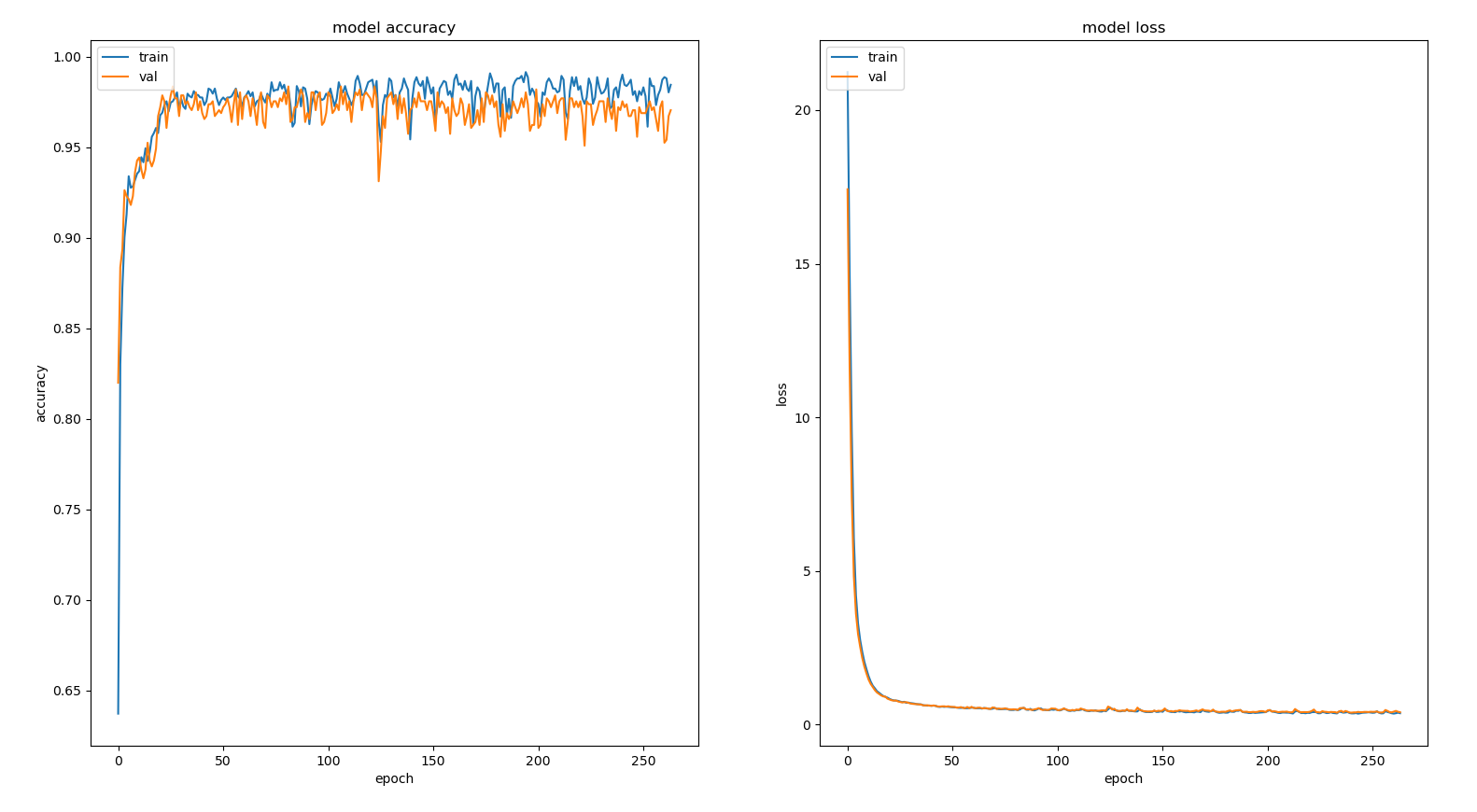## Posts

### Complete Classification ResultsFor a proper test of the trained neural network, we splitted our samples by users (instead of using the 0.7 / 0.3 approach). Training data: User 0 = 498 samples User 1 = 510 samples User 2 = 504 samples User 3 = 524 samples Total of 2035 samples (1425 for training + 610 for validation)   Test data: User 4 = 261 samples User 5 = 261 samples   Total of 522 samples  This way, we can ensure that all test samples were collected at a different time (with new force/torque sensor calibration) and by different users than the training samples. Learning Curves   Epoch 264/300 loss: 0.3699 - accuracy: 0.9846 val_loss: 0.4017 - val_accuracy: 0.9705   Using 1425 samples for training and 610 for validation   Confusion Matrices  Total test samples: 522 Correctly predicted: 507               Outputs Confidence Analysis          Legend:  Max confidence reached ( green line ) Mean of every confidences ( bold value ) Standard deviation (right below mean) Min confidence reached ( red line )    Accuracy